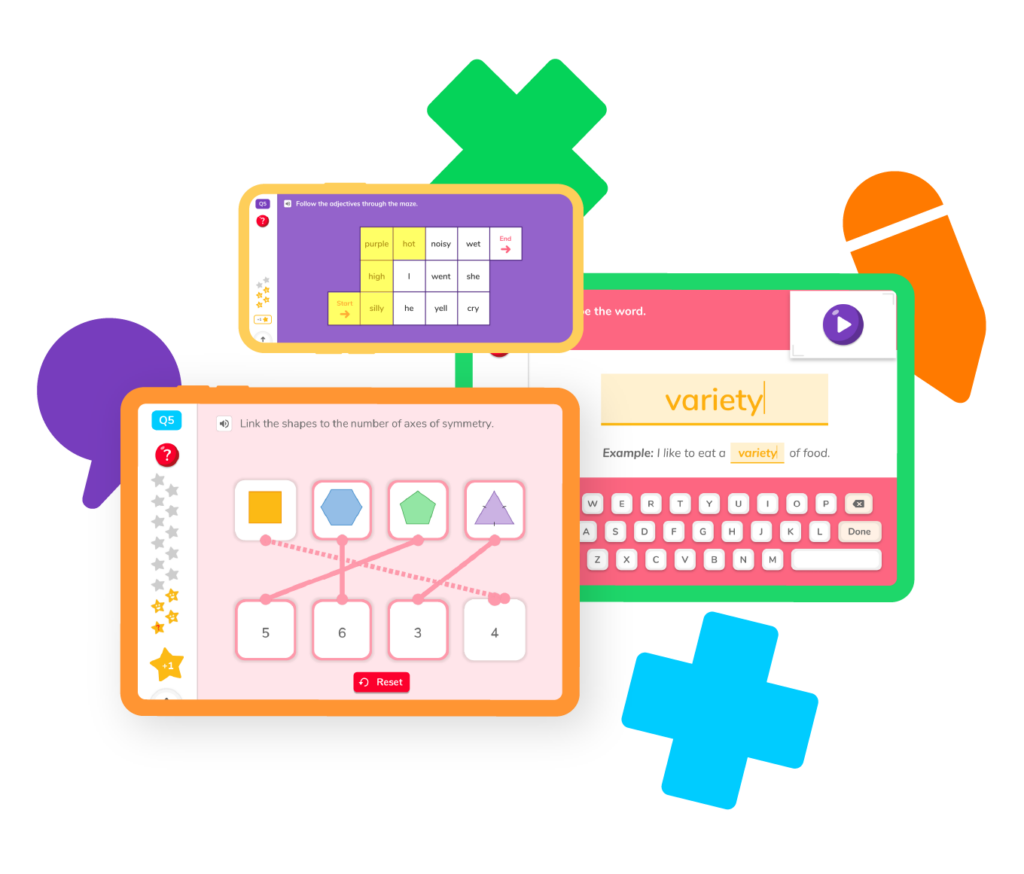# Learning Multiples and Factors

Everyone’s heard the age-old phrase: “learn those multiplication tables!” But there’s a lot more to multiples and factors than meets the eye. Whether students are looking for extra practice to keep their skills sharp or searching for helpful guides to help them better understand difficult concepts, DoodleMath has the resources, games, and worksheets you need to brush up on multiples, factors, and more.## Multiples and Factors resourcesWhether you’re identifying multiples, finding the least common factor, or simply trying to understand the properties of multiplication, DoodleMath has all the tools and resources you need to master multiples and factors.

## Multiples

See what happens when you multiply numbers together.

## Prime factorization

Break numbers down as far as you can with prime factorization.

## Properties of multiplication

Learn the rules of the multiplication road.

## Find the greatest common factor

Identify the largest factor all numbers in a given problem share.

## Find the least common multiple

Find the smallest number among all common multiples of a given number.

## Practice problems

Put your skills to the tests with practice problems that focus on multiples and factors.

## Multiples

See what happens when you multiply numbers together.

## Prime factorization

Break numbers down as far as you can with prime factorization.

## Properties of multiplication

Learn the rules of the multiplication road.

## Find the greatest common factor

Identify the largest factor all numbers in a given problem share.

## Find the least common multiple

Find the smallest number among all common multiples of a given number.

## Practice problems

Put your skills to the tests with practice problems that focus on multiples and factors.Multiples are numbers you get when you multiply a number by another number. For example, the multiples of 3 are 3, 6, 9, 12, and so on. You can keep adding 3 to get more multiples! So add 3 to 12, you get 15, add 3 to 15, you get 18, and on and on.

Factors are numbers that divide evenly into another number without leaving any remainder. For example, the factors of 12 are 1, 2, 3, 4, 6, and 12. When you divide 12 by these numbers, there won’t be any leftovers!

To find the multiples of a number, start with that number and keep adding it to itself. For example, if you want to find the multiples of 4, you start with 4 and add 4 to get 8, then add 4 again to get 12, and so on. You can keep going as far as you want!

To find the factors of a number, you need to think about what numbers you can divide it by without having any leftovers. Start with 1 and try dividing the number. If it divides evenly, then it’s a factor! Keep trying larger numbers until you reach the number itself. For example, to find the factors of 24, you would try dividing by 1, 2, 3, 4, 6, 8, 12, and 24.# Are you a parent, teacher or student?

Are you a parent or teacher?

## Hi there!

Book a chat with our team